Courses

# BITSAT Chemistry Test - 1

## 40 Questions MCQ Test BITSAT Subject Wise & Full Length Mock Tests | BITSAT Chemistry Test - 1

Description
This mock test of BITSAT Chemistry Test - 1 for JEE helps you for every JEE entrance exam. This contains 40 Multiple Choice Questions for JEE BITSAT Chemistry Test - 1 (mcq) to study with solutions a complete question bank. The solved questions answers in this BITSAT Chemistry Test - 1 quiz give you a good mix of easy questions and tough questions. JEE students definitely take this BITSAT Chemistry Test - 1 exercise for a better result in the exam. You can find other BITSAT Chemistry Test - 1 extra questions, long questions & short questions for JEE on EduRev as well by searching above.
QUESTION: 1

### Which of the following will not be soluble in sodium carbonate solution?

Solution: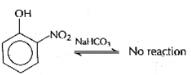While 2,4,6-trinitrophenol, benzoic acid and benzene sulphonic acid are soluble in NaHCO3.This reaction is possible in the forward direction if acid is more acidic than H2CO3.o-nitrophenol is less acidic than H2CO3.Hence, it is not soluble in sodium hydrogen carbonate.

QUESTION: 2

Solution:
QUESTION: 3

### Which of the following expressions gives the de-Broglie relationship?

Solution:
QUESTION: 4

The total number of electrons present in all the ρ-orbitals of bromine is

Solution:
QUESTION: 5

Point out incorrect statement about resonance

Solution:
QUESTION: 6

A compound possesses 8% sulphur by mass. The least molecular mass is

Solution:
QUESTION: 7

The compound with carbon uses only its sp3 hybrid orbitals for bond formation is

Solution: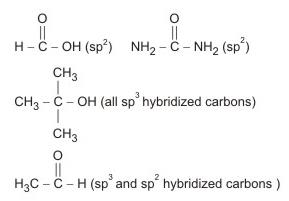QUESTION: 8

Which of the following gives maximum energy in metabolic process?

Solution:
QUESTION: 9

The main structure features of proteins is

Solution:
QUESTION: 10

Which of the following is the strongest nucleophile?

Solution:

A negatively charged nucleophile is always stronger than a neutral one

QUESTION: 11

Among the following the bond with highest bond dissociation energy is

Solution:
QUESTION: 12

The enthalpy change (∆H) for neutralisation of 1 M HCl by caustic soda in dilute solutions at 298 K is

Solution:
QUESTION: 13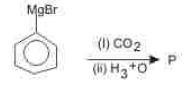In the above reaction , product P is

Solution:
QUESTION: 14

A and B are gaseous substances which react reversibly to give two gaseous substances C and D,accompanied by the liberation of heat.When the reaction reaches equilibrium,it is observed that Kp = Kc.The equilibrium cannot be disturbed by

Solution:
QUESTION: 15

The rate constant of first order reaction whose half life is 480 sec is

Solution:
QUESTION: 16

Which of the following elements has the lowest ionisation potential

Solution:

The ionisation potential increases from O to F to Ne, because of increasing nuclear charge. The ionisation potential is highest for Ne because of its stable (ns2 np6) electronic configuration. But the ionisation potential of O is lower than N although the nuclear charge of O is higher. This is due to the extra-stability of exactly half-filled 2p-orbitals of N.

QUESTION: 17

A system is said to be in thermodynamic equilibrium when it is in

Solution:
QUESTION: 18

The detergents which are most suitable for cleansing synthetic fibres, are

Solution:
QUESTION: 19

IUPAC name of Na₃[Co(NO₂)₆] is

Solution:

The IUPAC name of Na3[Co(N02)6] is sodium hexanitro cobaltate (III).

QUESTION: 20

Four successive members of the first row transition elements are listed below with their atomic no. Which of them is expected to have the highest third ionisation enthalpy?

Solution:
QUESTION: 21

Low spin complexes are generally formed by the elements of

Solution:
QUESTION: 22

The units of electrochemical equivalent are

Solution:
QUESTION: 23

Which of the following isomers will have the highest boiling point ?

Solution:
QUESTION: 24

When n-propyl iodide is heated with alcoholic KOH,one of the products is

Solution:
QUESTION: 25

The volume strength of 1.5 N H₂O₂ solution is

Solution:
QUESTION: 26

The process of separation of racemix mixture into its constitutes is called

Solution:
QUESTION: 27

The solubility of AgI in NaI solution is less than that in pure water because

Solution:
QUESTION: 28

How many monochlorobutanes will be possible on chlorination of n-butane?

Solution: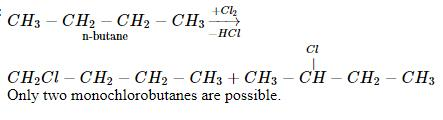QUESTION: 29

Of the following which is paramagnetic and has three electron bond in its structure

Solution:
QUESTION: 30

Which of the following is the strongest base?

Solution:
QUESTION: 31

In which one of the following compounds, the oxidation number of sulphur is the least

Solution:
QUESTION: 32

A metal X on heating in nitrogen gas gives Y, Y on treatment with H₂O gives a colourless gas which when passed through CuSO₄ soution gives a blue colour. Y is

Solution:
QUESTION: 33

What are the products formed when ammonia reacts with excess chlorine?

Solution:
QUESTION: 34

Glyptal polymer is obtained from glycol by reacting with

Solution:
QUESTION: 35

What are the products formed when Li2CO3 undergoes decomposition?

Solution:
QUESTION: 36

A solution of sodium metal in liquid ammonia is strongly reducing due to the presence of

Solution:
QUESTION: 37

The normality of a solution of NaOH; 100 ml. of which contains 4 g of NaOH is

Solution:
QUESTION: 38

The second order Bragg diffraction of x-rays with λ = 1 Å from a set of parallel planes in a metal occurs at an angle of 60o. The distance between the scattering planes in the crystal is

Solution:
QUESTION: 39

When 10 g of 90% pure limestone is heated completely, the volume (in litres) of carbon dioxide liberated at STP is

Solution: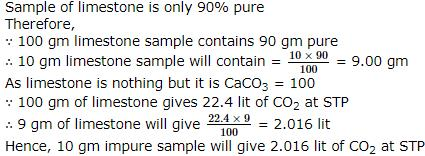QUESTION: 40

Surface tension of lyophilic sols is

Solution: